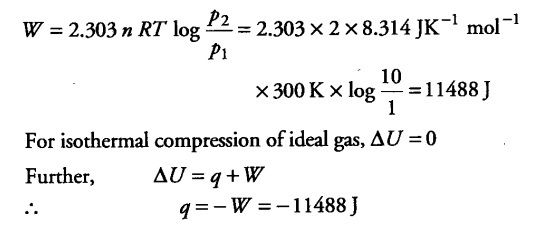# Two moles of an ideal gas initially at 27°C and one atmospheric pressure

Two moles of an ideal gas initially at 27°C and one atmospheric pressure are compressed isothermally and reversibly till the final pressure of the gas is 10 atm. Calculate q, W and ∆U for the process.

Here, n = 2 moles, T = 27° C = 300 K, { p }_{ 1 } =1 atm,
{ p }_{ 2 } =10 atm